###### A tutorial on Pandas apply, applymap, map, and transform
2022-06-23###### A complete guide on Pandas Grouping, Aggregating, and Transformation
2022-06-26

One of the foundational models for time series forecasting is the moving average model, denoted as MA(q). This is one of the basic statistical models that is a building block of more complex models such as the ARMA, ARIMA, SARIMA, and SARIMAX models. A deep understanding of the MA(q) is thus a key step prior to using more complex models to forecast intricate time series.

In this article, we first define the moving average process and explore its inner workings. We then use a dataset to apply our knowledge and use the ACF plot to determine the order of an MA(q) model.

## Defining the moving average process

A moving average process, or the moving average model, states that the current value is linearly dependent on the current and past error terms. Again, the error terms are assumed to be mutually independent and normally distributed, just like white noise.

A moving average model is denoted as MA(q) where q is the order. The model expresses the present value as a linear combination of the mean of the series (mu), the present error term (epsilon), and past error terms (epsilon). The magnitude of the impact of past errors on the present value is quantified using a coefficient denoted with theta. Mathematically, we express a general moving average process as follows:

The order q of the moving average model determines the number of past error terms that affect the present value. For example, if it is of order one, meaning that we have a MA(1) process, then the model is expressed as follows:

If we have a moving average process of order two, or MA(2), then we express the equation like this:

Hence, we can see how the order q of the MA(q) process affects the number of past error terms that must be included in the model. The larger q is, the more past error terms affect the present value. Therefore, it is important to determine the order of the moving average process in order to fit the appropriate model, meaning that if we have a second-order moving average process, then a second-order moving average model will be used for forecasting.

Notice that each value ofcan be thought of as a weighted moving average of the past few forecast errors. However, moving average models should not be confused with moving average smoothing. A moving average model is used for forecasting future values while moving average smoothing is used for estimating the trend cycle of past values.. Right: MA(2) with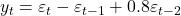. In both cases,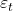is normally distributed white noise with mean zero and variance one.” width=”100%”>

Two examples of data from moving average models with different parameters.

The above figure shows some data from an MA(1) model and an MA(2) model. Left: MA(1) with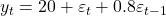. Right: MA(2) with. In both cases, εtεt is normally distributed white noise with mean zero and variance one. Changing the parameters θ1,…,θq results in different time series patterns. As with autoregressive models, the variance of the error term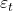will only change the scale of the series, not the patterns.

It is possible to write any stationary AR(p) model as an MA(∞) model. For example, using repeated substitution, we can demonstrate this for an AR(1) model: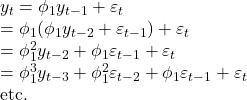Provided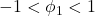, the value of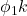will get smaller as k gets larger. So eventually we obtain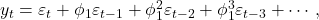an MA(∞) process.

The reverse result holds if we impose some constraints on the MA parameters. Then the MA model is called invertible. That is, we can write any invertible MA(qq) process as an AR(∞∞) process. Invertible models are not simply introduced to enable us to convert from MA models to AR models. They also have some desirable mathematical properties.

## Identifying the order of a moving average process

To identify the order of a moving average process, we follow the steps outlined below:

As usual, the first step is to gather the data. Then, we test for stationarity. In the event that our series is not stationary, we apply transformations, such as differencing, until the series is stationary. Then, we plot the ACF and look for significant autocorrelation coefficients. In the case of a random walk, we will not see significant coefficients after lag 0. On the other hand, if we see significant coefficients, then we must check if they become abruptly non-significant after some lag q. If that is the case, then we know that we have a moving average process of order q. Otherwise, we must follow a different set of steps to discover the underlying process of our time series.

Let’s put this in action using our data for the volume of sales of widgets for the XYZ Widget Company. The dataset contains 500 days of sales volume data starting on January 1, 2019. We will follow the set of steps outlined in figure 4.3 and determine the order of the underlying moving average process.

You can grab the dataset here. Note that the data is synthetic, as we rarely observe real-life time series that can be modeled with a pure moving average process. This dataset is thus for learning purposes.

The full source code is available here.

The first step is to gather the data. While this step was already done for you, this is a great time to load the data into a DataFrame using pandas and display the first five rows of data:

import pandas as p


You see that our volume of sales is in the column widget_sales. Note that the volume of sales is in units of thousands of US dollars.

We can then plot the data using the following piece of code:

import matplotlib.pyplot as plt
fig, ax = plt.subplots()
ax.plot(df.widget_sales)
ax.set_xlabel('Time')
ax.set_ylabel('Widget sales (k\$)')

plt.xticks(
[0, 30, 57, 87, 116, 145, 175, 204, 234, 264, 293, 323, 352, 382, 409, 439, 468, 498],
['Jan', 'Feb', 'Mar', 'Apr', 'May', 'Jun', 'Jul', 'Aug', 'Sep', 'Oct', 'Nov', 'Dec', '2020', 'Feb', 'Mar', 'Apr', 'May', 'Jun'])      #D
fig.autofmt_xdate()
plt.tight_layout()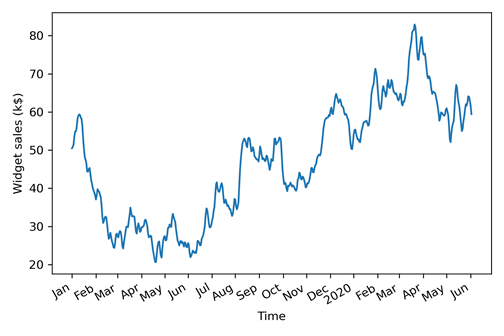Volume of widget sales for the XYZ Widget Company over 500 days, starting on January 1, 2019. Image by the author.

The next step is to test for stationarity. We intuitively know that the series is not stationary since there is an observable trend as see in the figure above. Still, we will use the ADF test to make sure. Again, we use the adfuller function from the statsmodels library and extract the ADF statistic and p-value. If the ADF statistic is a large negative number and the p-value is smaller than 0.05, then our series is stationary. Otherwise, we must apply transformations.

from statsmodels.tsa.stattools import adfuller


This results in an ADF statistic of -1.51 and a p-value of 0.53. Here, the ADF statistic is not a large negative number and the p-value is greater than 0.05. Therefore, our time series is not stationary and we must apply transformations to make it stationary.

In order to make our series stationary, we will try to stabilize the trend by applying a first-order differencing. We can do so by using the diff method from the numpy library. Remember that this method takes in a parameter n that specifies the order of differencing. In this case, because it is a first-order differencing, n will be equal to 1.

import numpy as np
widget_sales_diff = np.diff(df.widget_sales, n=1)


With a transformation applied to our series, we can test for stationarity again using the ADF test. This time, make sure to run the test on the differenced data stored in the widget_sales_diff variable.

ADF_result = adfuller(widget_sales_diff)


This gives an ADF statistic of -10.6 and a p-value of virtually 0. Therefore, with a large negative ADF statistic and a p-value much smaller than 0.05, we can say that our series is stationary.

Our next step is to plot the autocorrelation function. The statsmodels library conveniently includes the plot_acf function for us. We simply pass in our differenced series and specify the number of lags in the lags parameter. Remember that the number of lags determines the range of values on the x-axis.

from statsmodels.graphics.tsaplots import plot_acf
plot_acf(widget_sales_diff, lags=30);
plt.tight_layout()


The resulting ACF plot is shown below. We notice that there are significant coefficients after lag 0. In fact, they are significant up until lag 2. Then, they abruptly become non-significant as they remain in the shaded area of the plot. We can see some significance around lag 20, but this is likely due to chance, as the following coefficients are not significant.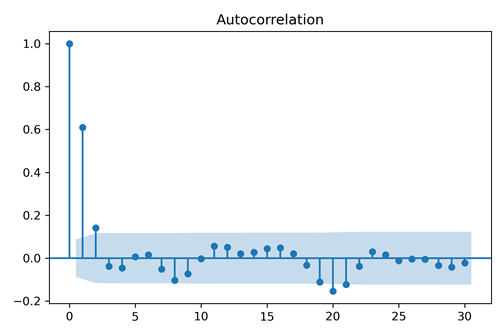ACF plot of the differenced series. Notice how coefficients are significant up until lag 2 before falling abruptly in the non-significance zone (shaded area) of the plot. We see some significant coefficients around lag 20, but this is likely due to chance since they are non-significant between lag 3 and 20 and after lag 20. Image by the author.

Since we have significant autocorrelation coefficients up until lag 2, this means that we have a stationary moving average process of order 2. Therefore, we can use a second-order moving average model, or MA(2) model, to forecast our stationary time series.

Thus, we can see how the ACF plot helps us determine the order of a moving average process. The ACF plot will show significant autocorrelation coefficients up until lag q, after which all coefficients will be non-significant. We can then conclude that we have a moving average process of order q or MA(q) process. In our case, working with the volume of widget sales, we discovered that the stationary process is a second-order moving average process since the ACF plot showed significant coefficients up until lag 2.

## Conclusion

In this article, we defined the moving average process and experienced how the ACF plot can be used to find the right order of the MA(q) model. This model can be used to forecast the time series.

Source:

https://towardsdatascience.com/defining-the-moving-average-model-for-time-series-forecasting-in-python-626781db2502

https://otexts.com/fpp2/MA.html##### Amir Masoud Sefidian
Machine Learning Engineer